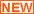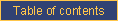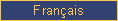homefeaturesDOCUMENTATIONpricingcontact ustechnical documentationCATI documentationcookbookrobotQuestionnaire structure

Permutation of questions

By default, CallWeb displays the questions in the order in which they are defined in the questionnaire. This default order can be affected by simple skips and complex skips. It can also be randomised via the permutation of questions.

CallWeb uses the # PERMUTATION instruction to define sets of consecutive questions which are to be displayed in random order. The syntax follows:

# Permutation NAME = VAR1 - VAR2 STEP=step LIMIT=limit LIKE=permutation

where

• NAME is the name of the permutation; any unique alphanumeric string is acceptable;
• VAR1 is the first variable to include on the permutation set;
• VAR2 is the last variable to include on the permutation set; obviously VAR2 must come after VAR1 in the questionnaire order;
• STEP is the number of variables in each block to be permutated (default = 1; can also be named PAS).
• LIMIT is the number of blocks of variables (blocks are defined by the STEP parameter) to display (default = all; can also be named LIMITE).
•LIKE (COMME in French) is the name of another permutation that will drive the order of the current permutation (default, none).

In the following example,

# Permutation 1 = Q5-Q10 STEP=2

Q6 always comes right after Q5, as will Q8 after Q7 and Q10 after Q9. However, the three pairs are offered in random order to respondents as in:

Q7-Q8, Q5-Q6, Q9-Q10
or
Q9-Q10, Q7-Q8, Q5-Q6

In the following example,

# Permutation 1 = Q5-Q10 STEP=1 LIMIT=2

Only two randomly selected questions in the series from Q5 to Q10 are displayed, in random order.

In the following example,

# Permutation 1 = Q5-Q14 STEP=2 LIMIT=2

Only two randomly selected blocks of two questions in the series from Q5 to Q14 are displayed. They could be Q7-Q8 and Q11-Q12, for example.

Display conditions affect which variables are displayed throughout the questionnaire, including in permutations.

Parallel permutationsSometimes, the order of a second set of questions must be the same as the random order of a first set of permutated questions. This is achieved by adding the LIKE= parameter to the second permutation definition followed by the name of the first permutation. For example:

# Permutation P1 = Q5-Q14 STEP=2 LIMIT=2
# Permutation P2 = Q15-Q24 LIKE=P1

Permutation P2 will always be presented in the same order as permutation P1. Note that the LIKE parameters do not supercede the STEP and LIMIT parameters. The number of items in the two permutations must be the same. Different display conditions may apply and modulate the number of items displayed in a given questionnaire but the order of those displayed will be the same.

Permutation within permutation: embedding permutations at any depth

All of the features described above can be implemented in permutations that are performed within permutated blocks. For example, in the following definitions:

# Permutation E001 = Q1-Q15 STEP=5
# Permutation I002 = Q1-Q5
# Permutation I003 = Q11-Q12
• one exterior permutation E001 entails three blocks of 5 questions which will appear in random order (e.g., sometimes Q1-Q5, then Q11-Q15, then Q6-Q10)
• one interior permutation I002 presents Q1 to Q5 in random order;
• another interior permutation I003 makes Q11 and Q12 appear in random order (e.g., an interior permutation does not need to use an entire block of the exterior permutation).

The questions of a permutation cannot overlap between blocks of the exterior permutation. Also, a permutation cannot include questions that are part of an exterior permutation and other questions that are not.

Note: permutation imbedding works only if the names of the permutations are chosen such that, in each case, the exterior permutation comes before the interior permutation, in alphabetical order of permutation names.

Such imbedding of permutations can be done at any depth level (e.g., permutations within permutations within permutations within ...).e-mail technical support!